##### Hot Keywords
Complex Engineering Systems Nonlinear Digital Twin PID Vehicle Prediction Fault Diagnosis

TopComplex Eng Syst 2021;1:8. 10.20517/ces.2021.05 © The Author(s) 2021.
Open Access Research Article

# Observer-based integral sliding mode control of nonlinear systems with application to single-link flexible joint robotics

School of Electronic and Information Engineering, Suzhou University of Science and Technology, Suzhou 215009, Jiangsu, China.

Correspondence Address: Dr. Baoping Jiang, School of Electronic and Information Engineering, Suzhou University of Science and Technology, No. 1 Kerui Road, Gaoxin District, Suzhou 215009, Jiangsu, China. E-mail: baopingj@163.com

Views:1426 | Downloads:232 | Cited:0 | Comments:0 | :499
Academic Editor: Hamid Reza Karimi | Copy Editor: Xing Zhou | Production Editor: Xing Zhou

## Abstract

This paper proposes the topics of sliding mode control for nonlinear Takagi-Sugeno systems based on a state observer with application to single-link flexible joint robotic. Firstly, a state observer relying on estimated premise variables is constructed, based on which we define an integral-type switching surface function on the estimation space. Secondly, by the equivalent control method, a sliding mode dynamics with an error system is obtained. Then, an adaptive variable structure controller is constructed to make sure that the predefined switching surface will be arrived in finite-time. Furthermore, stability analysis with an H performance is analyzed for the whole closed-loop system by linear matrix inequality condition. Finally, simulation study based on the robotics is conducted to confirm the validity of the proposed observer-based fuzzy controller.

## 1. INTRODUCTION

The research of nonlinear systems has always been the most popular topic in control theory and applications. Since no matter from internal and external perturbations or mechanism of dynamics, systems suffer from non-linearities is more common in practice. However, many traditional tools were good at tackling linear systems and invalid for nonlinear systems. Therefore, the issue of nonlinear control strategies comes to researchers' eyes, one of which was the Takagi-Sugeno (T-S) fuzzy approaches, which was viewed as a powerful mathematical method in dealing with complex nonlinear dynamics. Relying on a group of "IF-THEN" rules, the T-S fuzzy model provides accurate approximation of smooth nonlinear terms via fuzzy "blending" of local linear dynamics with the help of membership functions. Recently, fruitful research results have been proposed in literature by virtue of the T-S fuzzy approach. For example, the fault prognostics when the degradation phenomena exhibit nonlinear and time-varying dynamics by error based revolving T-S fuzzy model in; An input-output stabilization issue for time-delay systems via T-S fuzzy method was studied in; In, the fuzzy observer design and H controller designs for T-S fuzzy systems were investigated, for more details, see[5-7] and references therein.

On one hand, the system state components are not always available because of various limitations in practice, which means the analysis and feedback control of such systems based on observers is imperative. On the other hand, it happens that the system premise variables are the same with the state components, thus to achieve an effective sliding mode observer design by T-S fuzzy method, the estimated fuzzy dynamics combined with state-dependent premise variables must be considered in many practical problems. So far, a few pioneer works have taken efforts on this problem, for instance, the fuzzy state observer design in the sense uncertain input was proposed in. To the authors' knowledge, the issue of adaptive integral SMC for nonlinear T-S system with unmeasurable variables is interesting and an open issue to be studied.

In view of above discussion, this paper intents to investigate the issue of observer-based fuzzy integral SMC for nonlinear T-S systems. Based on the single-link flexible joint robotics models, a fuzzy model approach is introduced to obtain universal mathematical model. By designing an adaptive compensator in the state observer, an integral-type hyperplane function is proposed on the estimated space. The reachability of switching surface in finite-time is ensured by an adaptive sliding mode controller. A strict LMI condition is developed ensure stability and an H performance for the whole closed-loop dynamics.

Notations: In this paper, X > 0 depicts a positive definite matrix. || · || is a norm for Euclidean vector or spectral matrix. λmin(·) refers to minimum matrix eigenvalue. * denotes a matrix symmetric elements. He{P} represents PT + P.

## 2. PRELIMINARIES

Let's consider the following single-link flexible joint robotic dynamics:

in which ϑm(t) and ϑl(t) denote the angles of motor and link rotations, respectively. The corresponding angular velocities are denoted by ωm (t) and ωl(t). Jm is the inertia of the actuator and Jl means the inertia of the link. The following Table 1 shows the meaning of some other parameters.

Table 1

NOTATIONS

SymbolsMeaning
m (kg)Pointer mass
kl (N.m.V–1)Viscous friction coefficient
Bm (N.m.V–1)Amplifier gain

Now, denote θ1(t) = ϑm(t), θ2(t) = ωm(t), θ3(t) = ϑl(t) and θ4(t) = ωl(t). Then, following the method proposed in that under certain angle position, the function sin(θ3(t)) can be presented as

where β = 0.01/π is a constant and h1(θ3(t)) + h2(θ3(t)) = 1 with h1(θ3(t)), h2(x3(t)) ) [0,1]. Consequently, the functions h1(θ3(t)) and h2(θ3(t)) are solved as

It is easily seen in above functions that h1(θ3(t)) = 1 and h2(θ3(t)) = 0 if x3(t) is about 0 rad, h1(θ3(t)) = 0 and h2(θ3(t)) = 1 if θ3(t) is about π rad or –π rad. Therefore, in the state-space, the robotic system(1) can be presented by:

THEN

THEN

where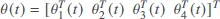and

More generally, by taking consideration of unknown local perturbations, the following universal model is considered:

Plant Rule i: IF x1(t) is Fi1 and x2(t) is Fi2 and ··· and xn(t) is Fin THEN

where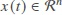is the state vector; x1 (t),..., xn (t) are also seen as the premise variables; Fij (i = 1,2..., r; j = 1,2..., n) are the fuzzy sets,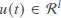is the control input;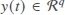is the controlled output, Ai, B and C are the system matrices with B has full column rank. The local unknown nonlinearity function f(x(t),t) is assumed to satisfy

with α > 0 and β > being unknown.

By fuzzy blending, the overall system is depicted as:

in which hi(x(t)) stands for the membership function described as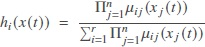with μij(xj(t)) being the grade of membership of xj(t) in μij. In addition, for any t > 0, it also meets that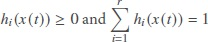.

In this paper, the purposes are to construct a fuzzy observer-based SMC strategy for the fuzzy system (3) such that the unknown local nonlinearity can be compensated by adaptive compensator and a prescribed H performance and good stability property can be obtained.

Remark 1 In the above, we proposed a single-link joint manipulator based system model to study the SMC design. In fact, we know that SMC can be combined with different algorithm to improve the its performance, for instance, the super twisting algorithm could avoid some disadvantages of SMC, therefore, it has significant potential application in the field such as knee exoskeleton and DC-DC converter, etc.

## 3. MIAN RESULTS

### 3.1. Fuzzy state observer design

In this section, due to the premise variables are also unmeasurable. Then, the following fuzzy state observer is constructed:

Plant Rule i: IF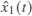is Fi1 and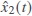is Fi2 and ··· and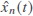is Fin

THEN

where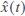and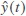correspondingly estimate the original state components and output. vs(t) is a designed compensator to attenuate unknown local perturbation. Li is the observer gain to be given in advance.

Similarly, the fuzzy observer (4) is depicted by

Let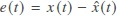be the estimated error. In view of systems (3) and (5), it obtains the following error dynamics:

where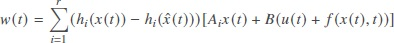is seen as disturbance satisfying w(t) ∈ L2[0, + ∞], from which it is obvious that w(t) = 0 if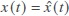.

### 3.2. Switching surface design

In this part, relying on the estimated component (5), an integral-type switching surface function is proposed in the following:

with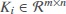to be designed.

Taking the systems (5) and (7) into consideration, it yields

When the switching surface s(t) = 0 is arrived, i.e.,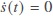, from which it obtains the following equivalent control variable

Then, combining (9) with (5), we have the sliding mode dynamics

Remark 2 By proposing the sliding mode observer (4) and switching surface function (7), we can see from the resulting sliding mode dynamic (10) that the sliding motion is totally insensitive to disturbance f(x(t), t), in addition, the disturbance can be attenuated by the adaptive compensator v(s), which verifies the advantages of SMC in dealing with nonlinear systems.

Up to now, we can conclude the aim of this work is to proposed a fuzzy sliding mode controller based on the fuzzy observer (5) in order to achieve two goals in the following:

• The systems (6) and (10) are stable in the presence of w(t) = 0;

• An H measurement

is satisfied with zero-initial condition, where γ is a positive scalar.

Now, as we can see, in order to attenuate the unknown local perturbation, vs(t) should be designed first. Based on the assumption made on f(x(t),t), we can employ two variables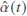and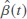to follow corresponding scalars α and β. Thus, it will be resulted in estimated errors, which are denoted by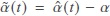and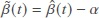, respectively.

So far, we can propose the compensator vs(t) below:

in which it is required that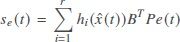with BTP = NC, ε is a positive scalar. In addition,andare designed by

with l1 and l2 are positive scalars to be selected in advance.

Remark 3 In view of above constrain BTP = NC, it is reasonable since we can see

Therefore, in the process of implementing the compensator, unavailable errors will be replaced by the fuzzy observer components and the original output variables.

### 3.3. SMC law design

In this part, we are to deal with the reachability of the predefined switching surface s(t) = 0, to this end, SMC scheme will synthesized to force the fuzzy observer state trajectories onto the switching surface s(t) = 0 in finite-time.

Theorem 1 By Proposing the switching surface function (7). The fuzzy SMC law constructed below can force the fuzzy observer state trajectories onto the switching surface s(t) = 0 in finite-time:

with ς > 0 is a tuning parameter,

Proof By considering the following Lyapunov function:

Then,

By substituting (14) into (16), it obtains

Therefore, we can conclude from (17) that the switching surface s(t) = 0 will be arrived in finite time. The proof is over.

### 3.4. H∞ performance analysis

In this part, we are going to give a sufficient condition to check if the systems (6) and (10) are stable and an H disturbance attenuation level γ is satisfied.

Theorem 2 For a selected γ > 0, the sliding mode dynamic (10) with the error system (6) are stable and satisfy an H performance, if there exist positive-definite matrix P > 0, Q > 0 and matrix Hi such that the condition holds in the following

and the controller gain is computed by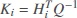and the state observer gain L1 is given such that Ai - BLiC is Hurwitz.

Proof Propose the following Lyapnuov function:

in which X > 0 and P > 0, Then, ones read

Taking the compensator vs(t) into consideration, it follows

Overall, it obtains

where η(t) = [xT(t) eT(t)]T,with

in which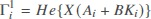,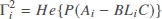, Now, denote Q = X-1, then pre-multiplying Γi with diag{Q, I} and post-multiplying Γi with diag{Q, I}. Letting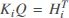, it obtains from the condition (18) that Γi < 0. Therefore, there is a scaler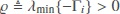such that

Therefore, for any t > 0,

So it yields

which means the system (10) is stable in the presence of w(t) = 0. Similarly, it can be proved that the error dynamic is also asymptotically stable.

Next, lets consider the H performance for the systems (6) and (10). It is obvious that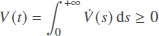in the sense of V(t) = 0. Therefore,

in which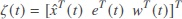,

Therefore, according to the Schur complement and the condition (is), it obtains that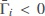, which means J < 0. Thus, we can drawn the conclusion the systems (6) and (10) are stable, and the H performance is also satisfied. This completes the proof.

Remark 4 In view of the constrain BTP2 = NC defined in the compensator is not solvable directly. The following linear condition is proposed, it is true that

Therefore, there is a scalar ϵ > 0 satisfies

In view of the Schur complement, we have

Therefore, the H performance issue we proposed before is now turned into by finding a global optimal solution in the following way:

with the condition in (is) satisfied simultaneously.

Therefore, the design of sliding mode observer in this paper is given as follows: For the plant (2), first, select appropriate gain matrices Li such that Ai – LiC is Hurwitz.]; Second, obtain controller gain matrices Ki by solving the inequality in (is) with constrain (27); Third, design compensator vs (t) with parameters gained from the second step; Last, we can design the sliding mode controller.

## 4. SIMULATION STUDY

Consider the single-link flexible joint robotic presented in model (1), the values of each parameter are shown in Table 2. Based on the fuzzy modeling approach proposed before, the system parameters, with g = 9.81, can be obtained as follows:

Table 2

SYSTEM PARAMETERS

Parameter(Units)Value
m(kg)2.1×10-3
h(m)1.5×10-1
kj(N·m·V-1)8.0 ×10-2
Bm (N·m·V-1)4.6 × 10-3
Jm3.7 × 10-3
Jl9.23×10-3

By setting the attenuation level γ = 3.5. Then, solving the LMI condition in remark 2 with the condition (18), where L1= L2= 10. Then, we have the following optimal solutions:

N = 0.0654, ϵ = 5.5473 ×10-4.

Therefore, the controller gain matrices can be computed as

With the help of above parameters, the simulation is performed in the following. Firstly, the initial conditions of original system and observer system are provided as x(t) = [0.10.1 –0.1 –0.1]T and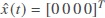. The unknown disturbance is assumed to be f(x(t)) = 0.01 sin(x1(t)), in the implementation of the adaptive compensator and fuzzy controller, relevant parameters are given as l1= l2= 0.01, ε = 0.01 and ς = 0.01. Besides, the switching signals sgn(s(t)) will be changed by s(t)/(||s(t)|| + 0.01) to remove chattering effect. Consequently, the simulation outcomes are shown in Figure 1-Figure 7. Figure 1 shows the trajectory changes of the original system under control; Figure 3 gives the trajectory changes of the observer system; Figure 3 depicts the switching surface function; Figure 6 represents the controller input; The value for adaptive gains are given in Figure 5 and Figure 6, respectively From those figures, it knows that the proposed control strategy achieved satisfactory performance. In addition, in order to demonstrate the superiority of the proposed controller over traditional state feedback controller u(t) = Kx(t) with the same gains obtained above, the following Figure 7 shows that the state is no longer stable with the disturbance getting stronger, i.e., f (x(t)) = 0.2 sin(x1 (t)).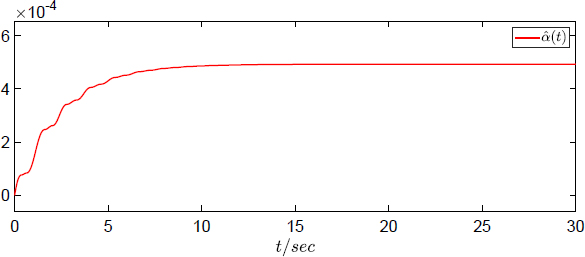Figure 5. Estimated value for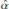.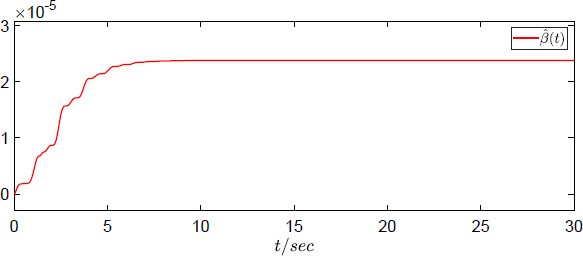Figure 6. Estimated value for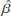.

Remarks 5 As we know, the chattering effect is always unavoidable by employing traditional SMC. Several methods have been proposed to deal with this paper, one of which was the approach proposed above by replace switching signals with smooth ones. Reducing chattering effect is an interesting issue that worths further investigation in the future.

## 5. CONCLUSION

In this paper, the topic of T-S fuzzy model-based state observer design for SMC of nonlinear systems with application to robotics model has been investigated. Firstly a state observer relying on estimated premise variables was constructed, based on which an integral switching surface has been developed. Secondly, sliding mode dynamics was derived through equivalent theory. Then, the arrival of switching surface was ensured by designing an adaptive sliding mode controller. Furthermore, stability analysis with an H performance were undertaken for the resulting systems. Finally, simulation study based on the robotics model has been conducted to confirm the validity of the fuzzy controller. In the near future, our attention will be focused on proposing more advanced control strategy to n-link robotic manipulator in terms of robustness, computation, operation cost and system limitation etc.

## DECLARATIONS

Authors' contributions

Made substantial contributions to supervision, writing, review, editing and methodology: Jiang B Performed writing-original draft, software, validation and visualization: Liu Q, Cai Z, Chen J

Availability of data and materials

Not applicable.

This work is supported by The National Natural Science Foundation of China under grant 62003231; partially supported by The Natural Science Foundation of Jiangsu Province under grant no. BK20200989, in part by the NCF for colleges and universities in Jiangsu Province under grant 20KJB120005, in part by Anhui Province Key Laboratory of Intelligent Building and Building Energy Saving under grant IBES2020KF01, and in part by The China Postdoctoral Science Foundation (2021M692369).

Conflicts of interest

All authors declared that there are no conflicts of interest.

Ethical approval and consent to participate

Not applicable.

Consent for publication

Not applicable.

## References

• 1. Takagi T, Sugeno M. Fuzzy identification of systems and its applications to modeling and control. IEEE Trans. Syst., Man, Cybern. B, Cybern 1985;15:116-32.

DOI
• 2. Camargos MO, Palhares RM, Bessa I, Cosme LB, Angelo MFSV. Data-driven prognostics of rolling element bearings using a novel error based evolving Takagi–Sugeno fuzzy model. Applied Soft Computing 2020;96:106628.

• 3. Zhao L, Gao H, Karimi HR. Robust stability and stabilization of uncertain T–S fuzzy systems with time-varying delay: an input–output approach. IEEE Transactions on Fuzzy Systems 2012;21:883-97.

• 4. Liu X, Zhang Q. New approaches to H controller designs based on fuzzy observers for TS fuzzy systems via LMI. Automatica 2003;39:1571-82.

DOI
• 5. Lin C, Wang Q, Lee TH. Improvement on observer-based H control for T-S fuzzy systems. Automatica 2005;41:1651-6.

• 6. Datta R, Dey R, Bhattacharya B. Improved delay-range-dependent stability condition for T-S fuzzy systems with variable delays using new extended affine Wirtinger inequality. Int J Fuzzy Syst 2020;22:985-98.

DOI
• 7. Coutinho PH, Araujo RF, Nguyen AT, Palhares RM. A multiple-parameterization approach for local stabilization of constrained Takagi-Sugeno fuzzy systems with nonlinear consequents. Information Sciences 2020;506:295-307.

DOI
• 8. Emelyanov SV. Variable Structure Control Systems Nauka, Moscow: 1967.

• 9. Tan S, Lai YM, Tse CK. Sliding mode control of switching power converters: techniques and implementation. CRC press; 2018.

• 10. Baek J, Jin M, Han S. A new adaptive sliding-mode control scheme for application to robot manipulators. IEEE Transactions on Industrial Electronics 2016;63:3628-37.

DOI
• 11. Azar A, Fernando E., Serrano FE. Adaptive sliding mode control of the Furuta pendulum. Advances and applications in sliding mode control systems. Springer, Cham; 2015. pp. 1-42.

• 12. Wang Y, Gao Y, Karimi HR, Shen H, Fang Z. Sliding mode control of fuzzy singularly perturbed systems with application to electric circuit. IEEE Transactions on Systems, Man, and Cybernetics: Systems 2018;48:1667-75.

DOI
• 13. Naghmeh M, Mehdi R S, Mahmood G. Robust control design for air breathing proton exchange membrane fuel cell system via variable gain second-order sliding mode. Energy Science & Engineering 2018;6:126-43.

DOI
• 14. Pan Y, Yang C, Pan L, Yu H. Integral sliding mode control: performance, modification, and improvement. IEEE Transactions on Industrial Informatics 2017;14:3087-96.

DOI
• 15. Gao Y, Liu J, Sun G, Liu M, Wu L. Fault deviation estimation and integral sliding mode control design for Lipschitz nonlinear systems. Systems & Control Letters 2019;123:8-15.

DOI
• 16. Jiang B, Gao C. Decentralized adaptive slidingmode control of large-scale semi-Markovian jump interconnected systems with dead-zone input. IEEE Transactions on Automatic Control 2021:1-1.

DOI
• 17. Jiang B, Karimi HR, Yang S, Gao C, Kao Y. Observer-based adaptive sliding mode control for nonlinear stochastic Markov jump systems via T-S fuzzy modeling: applications to robot arm model. IEEE Transactions on Industrial Electronics 2021;68:466-77.

DOI
• 18. Qi W, Gao X, Ann CK, Cao J, Cheng J. Fuzzy integral sliding-mode control for nonlinear semi-Markovian switching systems with application. IEEE Transactions on Systems, Man, and Cybernetics: Systems 2020:1-10.

DOI
• 19. Mani P, Joo Y. Fuzzy logic-based integral sliding mode control of multi-area power systems integrated with wind farms. Information Sciences 2021;545:153-69.

DOI
• 20. Norsahperi NMH, Danapalasingam KA. An improved optimal integral sliding mode control for uncertain robotic manipulators with reduced tracking error, chattering, and energy consumption. Mechanical Systems and Signal Processing 2020;142:106747.

DOI
• 21. Jia S, Shan J. Continuous integral sliding mode control for space manipulator with actuator uncertainties. Aerospace Science and Technology 2020;106:106192.

DOI
• 22. Teng L, Gull MA, Bai S. PD-based fuzzy sliding mode control of a wheelchair exoskeleton robot. IEEE/ASME Transactions on Mechatronics 2020;25:2546-55.

DOI
• 23. Roy S, Baldi S, Fridman L M. On adaptive sliding mode control without a priori bounded uncertainty. Automatica 2020;111:108650.

DOI
• 24. Huang AC, Chen YC. Adaptive sliding control for single-link flexible-joint robot with mismatched uncertainties. IEEE Transactions on Control Systems Technology 2004;12:770-5.

DOI
• 25. Rsetam K, Cao Z, Man Z. Hierarchical sliding mode control applied to a single-link flexible joint robot manipulator[C]// 2016 International Conference on Advanced Mechatronic Systems (ICAMechS). IEEE 2016; doi: 10.1109/ICAMECHS.2016.7813495.

DOI
• 26. Chen Y, Guo B. Sliding mode fault tolerant tracking control for a single-link flexible joint manipulator system. IEEE Access, 2019;PP:1-1.

DOI
• 27. Chadli M, Karimi HR. Robust observer design for unknown inputs Takagi-Sugeno models. IEEE Transactions on Fuzzy Systems 2012;21:158-64.

DOI
• 28. Saad W, Sellami A, Garcia G. H-Sliding mode control of one-sided Lipschitz nonlinear systems subject to input nonlinearities and polytopic uncertainties. ISA Transactions 2019;90:19-29.

DOIPubMed
• 29. Tanaka K, Kosaki T. Design of a stable fuzzy controller for an articulated vehicle. IEEE Transactions on Systems, Man, and Cybernetics: PartB(Cybern.) 1997;27:552-8.

DOIPubMed
• 30. Rakhtala S. M. Adaptive gain super twisting algorithm to control a knee exoskeleton disturbed by unknown bounds. International Journal of Dynamics and Control 2020:1-16.

DOI
• 31. Rakhtala S M, Casavola A. Real time voltage control based on a cascaded super twisting algorithm structure for DC-DC converters. IEEE Transactions on Industrial Electronics, 2021;PP:1-1.

DOI
• 32. Zhai J, Xu G. A novel non-singular terminal sliding mode trajectory tracking control for robotic manipulators. IEEE Transactions on Circuits and Systems II: Express Briefs 2021;68:391-5.

DOI

OAE Style

Liu Q, Cai Z, Chen J, Jiang B. Observer-based integral sliding mode control of nonlinear systems with application to single-link flexible joint robotics. Complex Eng Syst 2021;1:8. http://dx.doi.org/10.20517/ces.2021.05

AMA Style

Liu Q, Cai Z, Chen J, Jiang B. Observer-based integral sliding mode control of nonlinear systems with application to single-link flexible joint robotics. Complex Engineering Systems. 2021; 1(2):8. http://dx.doi.org/10.20517/ces.2021.05

Chicago/Turabian Style

Liu, Qi, Zhenyang Cai, Jie Chen, Baoping Jiang. 2021. "Observer-based integral sliding mode control of nonlinear systems with application to single-link flexible joint robotics" Complex Engineering Systems. 1, no.2: 8. http://dx.doi.org/10.20517/ces.2021.05

ACS Style

Liu, Q.; Cai Z.; Chen J.; Jiang B. Observer-based integral sliding mode control of nonlinear systems with application to single-link flexible joint robotics. Complex. Eng. Syst. 20211, 8. http://dx.doi.org/10.20517/ces.2021.05

Views
14260